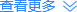#### 您所在的位置： 北京时间> 亚洲时差查询> 中国时差查询> 北京时差查询

2020年09月25日 星期五 17:06:36

• 时差：无
• 时差：无
• 时差：无
• 时差：无
• 时差：无
• 时差：无
• 时差：无
• 时差：无
• 时差：无
• 时差：无
• 时差：无
• 时差：无
• 时差：无
• 时差：无
• 时差：无
• 时差：无
• 时差：无
• 时差：无
• 时差：无
• 时差：无
• 时差：无
• 时差：无
• 时差：无
• 时差：无
• 时差：无
• 时差：无
• 时差：无
• 时差：无
• 时差：无
• 时差：无

[中国] xian 西安

• 时差：无
• 时差：无
• 时差：无
• 时差：无
• 时差：无

[中国] lasa 拉萨

• 时差：无
• 时差：无
• 时差：无
• 时差：无
• 时差：无
• 时差：无
• 时差：无
• 时差：无
• 时差：无
• 时差：无
• 时差：无
• 时差：无
• 时差：无
• 时差：无
• 时差：无
• 时差：无
• 时差：无
• 时差：无
• 时差：无

[中国] jixi 鸡西

• 时差：无
• 时差：无
• 时差：无
• 时差：无
• 时差：无
• 时差：无
• 时差：无
• 时差：无
• 时差：无
• 时差：无
• 时差：无
• 时差：无
• 时差：无
• 时差：无
• 时差：无
• 时差：无
• 时差：无
• 时差：无
• 时差：无
• 时差：无
• 时差：无
• 时差：无
• 时差：无
• 时差：无
• 时差：无
• 时差：无
• 时差：无
• 时差：无
• 时差：无
• 时差：无
• 时差：无
• 时差：无
• 时差：无
• 时差：无
• 时差：无
• 时差：无
• 时差：无
• 时差：无
• 时差：无
• 时差：无
• 时差：无
• 时差：无
• 时差：无
• 时差：无
• 时差：无
• 时差：无
• 时差：无
• 时差：无
• 时差：无
• 时差：无
• 时差：无
• 时差：无
• 时差：无
• 时差：无
• 时差：无
• 时差：无
• 时差：无
• 时差：无
• 时差：无
• 时差：无
• 时差：无
• 时差：无
• 时差：无
• 时差：无
• 时差：无
• 时差：无
• 时差：无
• 时差：无
• 时差：无
• 时差：无
• 时差：无
• 时差：无
• 时差：无
• 时差：无
• 时差：无
• 时差：无
• 时差：无
• 时差：无
• 时差：无
• 时差：无

[中国] zibo 淄博

• 时差：无
• 时差：无
• 时差：无
• 时差：无
• 时差：无
• 时差：无
• 时差：无
• 时差：无

[中国] heze 菏泽

• 时差：无
• 时差：无
• 时差：无
• 时差：无
• 时差：无
• 时差：无
• 时差：无
• 时差：无
• 时差：无
• 时差：无
• 时差：无
• 时差：无
• 时差：无
• 时差：无
• 时差：无
• 时差：无
• 时差：无
• 时差：无
• 时差：无

[中国] hami 哈密

• 时差：无
• 时差：无
• 时差：无
• 时差：无
• 时差：无
• 时差：无
• 时差：无
• 时差：无
• 时差：无

[中国] ali 阿里

• 时差：无
• 时差：无
• 时差：无
• 时差：无
• 时差：无
• 时差：无
• 时差：无
• 时差：无
• 时差：无
• 时差：无
• 时差：无
• 时差：无
• 时差：无
• 时差：无
• 时差：无
• 时差：无
• 时差：无
• 时差：无
• 时差：无
• 时差：无
• 时差：无
• 时差：无
• 时差：无
• 时差：无
• 时差：无
• 时差：无
• 时差：无
• 时差：无
• 时差：无
• 时差：无
• 时差：无
• 时差：无
• 时差：无
• 时差：无
• 时差：无
• 时差：无

[中国] hebi 鹤壁

• 时差：无
• 时差：无
• 时差：无
• 时差：无
• 时差：无
• 时差：无
• 时差：无
• 时差：无
• 时差：无
• 时差：无
• 时差：无
• 时差：无
• 时差：无
• 时差：无
• 时差：无
• 时差：无
• 时差：无
• 时差：无
• 时差：无
• 时差：无
• 时差：无
• 时差：无
• 时差：无
• 时差：无
• 时差：无
• 时差：无
• 时差：无
• 时差：无
• 时差：无
• 时差：无
• 时差：无
• 时差：无
• 时差：无
• 时差：无
• 时差：无
• 时差：无
• 时差：无
• 时差：无

[中国] wuhu 芜湖

• 时差：无
• 时差：无
• 时差：无
• 时差：无
• 时差：无
• 时差：无
• 时差：无
• 时差：无
• 时差：无
• 时差：无
• 时差：无
• 时差：无

[中国] luan 六安

• 时差：无
• 时差：无
• 时差：无
• 时差：无
• 时差：无
• 时差：无
• 时差：无
• 时差：无
• 时差：无
• 时差：无
• 时差：无
• 时差：无
• 时差：无
• 时差：无
• 时差：无
• 时差：无
• 时差：无
• 时差：无
• 时差：无
• 时差：无
• 时差：无
• 时差：无
• 时差：无
• 时差：无
• 时差：无
• 时差：无
• 时差：无
• 时差：无
• 时差：无
• 时差：无
• 时差：无
• 时差：无
• 时差：无
• 时差：无
• 时差：无
• 时差：无
• 时差：无
• 时差：无
• 时差：无
• 时差：无
• 时差：无
• 时差：无
• 时差：无
• 时差：无
• 时差：无
• 时差：无
• 时差：无
• 时差：无
• 时差：无
• 时差：无
• 时差：无
• 时差：无
• 时差：无
• 时差：无
• 时差：无
• 时差：无
• 时差：无

[中国] yaan 雅安

• 时差：无
• 时差：无
• 时差：无
• 时差：无
• 时差：无
• 时差：无
• 时差：无
• 时差：无
• 时差：无
• 时差：无
• 时差：无
• 时差：无
• 时差：无
• 时差：无
• 时差：无
• 时差：无
• 时差：无
• 时差：无
• 时差：无
• 时差：无
• 时差：无
• 时差：无
• 时差：无
• 时差：无
• 时差：无
• 时差：无
• 时差：无

[中国] yuxi 玉溪

• 时差：无

[中国] puer 普洱

• 时差：无
• 时差：无
• 时差：无
• 时差：无
• 时差：无
• 时差：无
• 时差：无
• 时差：无
• 时差：无
• 时差：无
• 时差：无
• 时差：无
• 时差：无
• 时差：无
• 时差：无
• 时差：无
• 时差：无
• 时差：无
• 时差：无
• 时差：无
• 时差：无
• 时差：无
• 时差：无
• 时差：无
• 时差：无
• 时差：无
• 时差：无
• 时差：无
• 时差：无
• 时差：无
• 时差：无
• 时差：无
• 时差：无
• 时差：无